Name:    Unit 5-Bonding Practice Test

Matching

Match each item with the correct statement below.
 a. diatomic d. cation b. binary compound e. anion c. polyatomic ion

1.

a positively charged ion, metals form these.

2.

a negatively charged ion, nonmetals form these

3.

a molecule made of two identical atoms like H2

4.

compound composed of two different elements.

5.

group of atoms that are bonded together and carry a + or - charge.

Match each item with the correct statement below.
 a. covalent e. valence electrons b. octet rule f. coordination number c. ionic bond g. metallic bond d. electron dot diagram

6.

are found in the outermost energy level of an atom

7.

a depiction of valence electrons around the symbol of an element

8.

Atoms will gain lose or share electrons in order to be like the closest a noble gas.

9.

the force of attraction binding oppositely charged ions together

10.

The type of bond formed by two nonmetals.

Multiple Choice
Identify the choice that best completes the statement or answers the question.

11.

Which of the following formulas represents a compound whose molecules contain six shared electrons?
 a. :NºN: b. O=O=O c. O3 d. SO3

12.

Which of the following is NOT a characteristic property of ionic compounds?
 a. They conduct electricity when dissolved in water. b. They form crystals. c. They have high melting points. d. They have low melting points.

13.

Ionic compounds are held together by a force best compared to________________?
 a. electronic repulsion c. both a and b b. magnetic attraction d. neither a or b

14.

The rule that sates that elements will gain, lose or share electrons in order to have a full outer shell is called?
 a. The Rule of 2 b. Foster’s rule c. None of these d. Octet rule

15.

In an electron dot diagram, the dots are used to represent
 a. protons c. neutrons b. all electrons. d. valence electrons.

16.

What is the chemical name for the compound with the formula Na2S?
 a. lithium oxide c. sodium fluoride b. sodium sulfide d. magnesium sulfide

17.

A chemical bond that occurs when atoms share electrons is a(n) ____ bond.
 a. polyatomic b. covalent c. magnetic d. ionic

18.

A single covalent bond contains _____  electrons?
 a. 2 b. 4 c. 1 d. 0

19.

A double covalent bond contains _____  electrons?
 a. 6 b. 0 c. 2 d. 4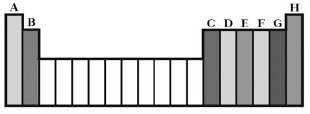20.

How many valence electrons do MOST Noble Gases have (column H)?
 a. 7 b. 4 c. 0 d. 8

21.

How many single covalent bonds can halogens form (Column G)?
 a. 1 b. 4 c. 3 d. 2

22.

The chemical formula for water, a covalent compound, is H2O. This formula is an example of a(n)
 a. molecular formula. c. Lewis structure. b. ionic formula. d. formula unit.

23.

How many covalent bonds can oxygen form?
 a. 3 b. 2 c. 4 d. 1

24.

What will happen to Magnesium , Mg when it bonds?
 a. gain 2 electrons c. gain 2 protons b. gain 6 electrons d. lose 2 electrons

25.

What molecular shape does the compound CCl4 have?
 a. octahedral b. trigonal planar c. bent d. tetrahedral

26.

Which element can never have atoms with eight valence electrons even when it forms bonds?
 a. carbon b. nitrogen c. oxygen d. hydrogen

27.

In what form can an ionic compound conduct electricity?
 a. when warmed slightly c. as a crystal b. as a solid d. when dissolved in water

28.

How many electrons are needed in the outer energy level of Hydrogen, H for the atom to be chemically stable?
 a. 0 b. 4 c. 2 d. 1

29.

The kinds and the exact number of atoms of each element in a unit of the compound can be shown in a ____.
 a. chemical symbol c. chemical formula b. superscript d. subscript

30.

What will happen to Oxygen, O when it bonds?
 a. lose 6 electrons c. lose 2 protons b. lose 2 electrons d. gain 2 electrons

31.

How many chloride ions (Cl1-) are needed to balance out the magnesium ion (Mg1+) create the compound magnesium chloride (MgCl2)?
 a. 1 b. 2 c. 4 d. 3

32.

What is the formula for dinitrogen trioxide?
 a. N2O3 b. NO3 c. N2O6 d. Ni2O3

33.

In the compound MgCl2, the subscript 2 indicates that
 a. the chloride ion is twice the size of the magnesium ion. b. magnesium and chlorine form a double covalent bond. c. there are two chloride ions for each magnesium ion. d. there are two magnesium ions for each ion of chlorine

34.

In the name carbon dioxide, the prefix of the second word indicates that a molecule of carbon dioxide contains
 a. two oxygen atoms. c. a polyatomic ion. b. two carbon atoms. d. an ionic bond.

35.

What is the correct Lewis structure for hydrogen chloride, HCl?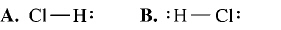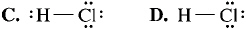a. A b. B c. C d. D

36.

You see a structural formula in which the symbols for elements are connected by a long dash. You can assume that the chemical bonds in the compound are
 a. covalent. b. ionic. c. unstable. d. metallic.

37.

The sum of the charges in a neutral compound is always ____.
 a. a positive number c. a negative number b. zero d. one

38.

If two covalently bonded atoms are identical, the bond is
 a. polar covalent. c. coordinate covalent. b. nonpolar covalent. d. dipole covalent.

39.

Why do the noble gases NOT form compounds readily?
 a. They have no electrons. b. Their outer energy levels are completely filled with electrons. c. They have seven electrons in the outer energy levels. d. They have empty outer energy levels.

40.

How many single covalent bonds can carbon form?
 a. 2 b. 3 c. 1 d. 4

41.

Which is the correct name for the compound Na3P?
 a. Sodium Phosphide c. Sodium Triphosphide b. Sodium Phosphite d. Sodium Phosphate

42.

What is the correct formula for the compound formed by Mg+2 and O-2?
 a. OMg c. MgO2 b. Mg2O d. MgO

43.

Study the electron dot diagrams below for lithium, carbon, fluorine, and neon and choose the correct statement below.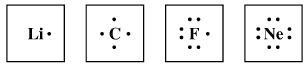a. Neon will bond with eight lithium’s to form an ionic bond. b. Carbon will bond with four lithium’s to form a covalent bond. c. Lithium will lose it’s only valence electron to fluorine to form a covalent bond. d. Lithium will lose it’s only valence electron and fluorine will accept it to form an ionic bond.

44.

A chemical bond that occurs when atoms share electrons EQUALLY is a(n) ____ bond.
 a. nonpolar b. polar c. polyatomic d. ionic

45.

Which of the following compounds is covalent compound?
 a. CaF2 b. MgCl2 c. H2O d. Na2S

46.

What kind of chemical bond is formed when a transfer of electrons occurs?
 a. covalent b. magnetic c. ionic d. hydrate

47.

Bonds that form between two nonmetals are usually
 a. covalent. b. ionic. c. impossible. d. weak.

48.

In the 4 molecules: N2, O2, HCl, and F2, which compound would be polar?
 a. HCl b. F2 c. O2 d. N2

49.

The formation of an ionic bond involves the
 a. transfer of positrons. c. transfer of electrons. b. transfer of neutrons. d. transfer of protons.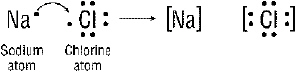Figure 20-1

50.

What is the name of the Figure above?
a.   monosodium monochloride                  c.   sodium chloride
b.   sodium monchlorine                        d.   sodium monochloride

51.

What charges are on the sodium (Na) and the chlorine ion (Cl) after the reaction in Figure 20-1(in the order that they are shown)?
a.   1+ and 1-            b.   0 and 1-            c.   1- and 1+             d.   0 and 0

52.

What happened during the reaction in Figure 20-1?
a.   Sodium gained an electron                        c.   Chlorine lost 7 electrons
b.   Sodium lost an electron                              d.   nothing happened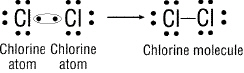Figure 20-2

53.

How is the bond formed in Figure 20-2 above?
a.  both chlorines share electrons.            c.   both chlorines lose their electrons.
b.  one chlorine takes all of the electrons.      d.   there is no bond formed.

54.

Do these on the back of your scantron! (7 points)
Using electron dot diagrams show how bonds are formed for:
a. Use arrows to show a transfer of electrons then draw the resulting ions to show the dot formation for:  MgF2.
Be sure to include the electron dots, the ion charges and which noble gas EACH is trying to be like.

b. Use circles to show sharing and then redraw the structure with dashes to   show the formation for: CH4

H

H      C     H

H
THE ATOMS ARE ARRANGED FOR YOU ALREADY.